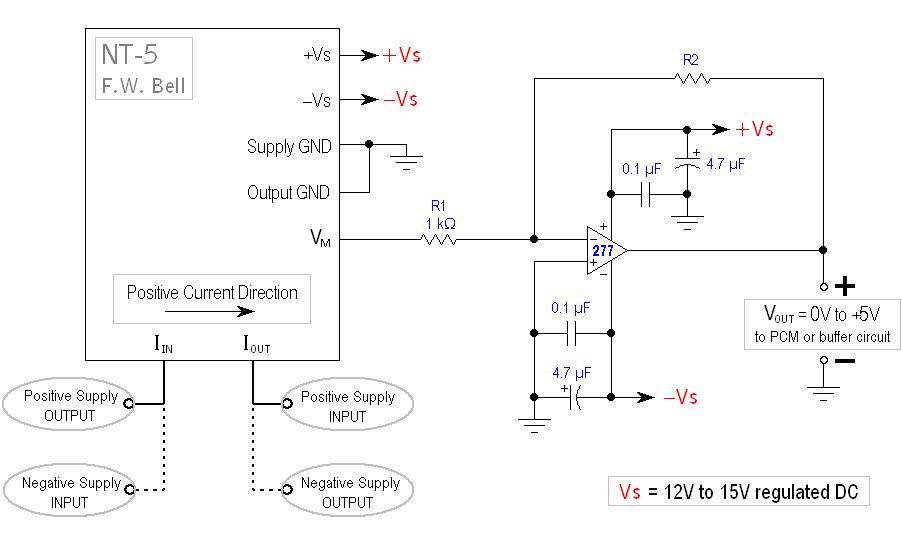Introduction: (written 9-4-06)

Designed to monitor current consumption of various circuit board trays on an atmospheric sounding-rocket payload for experimental study.

Support Module Current Sensors

Devin R Ott
Design - completed in October 2004

The supply currents of each device will be measured by the NT-5 magneto-resistive current sensors from F.W. Bell technologies.  These current-to-voltage converters have a nominal current rating of ±5 amps, and a corresponding nominal output of ±2.5 volts.  In other words, the currents sensors output:   VM=1/2IIN

Now that we have a voltage (VM) proportional to the supply current, we can employ an op-amp to fit our desired voltage range into the 0 to 5 volt window required for the PCM.  For example, a 0-100mA measurement range would create a 0-50mV output range, requiring an amplifier gain of 100V/V before going to the PCM.

The circuit below was designed to represent a current range (0 to IMAX) as a DC voltage range (0 to 5 volts) depending upon the value of the feedback resistor R2.

To measure (0 to IMAX) set:      R2 =10,000÷IMAX

To calculate IIN from the final output voltage (VOUT):       IIN = (VOUT÷5)×IMAXAs you may have noticed, the measured currents of both + and – supplies will be opposing the Positive Current Direction specified on the NT-5 sensor.  In other words, the sensor will be measuring negative current, and thus outputting a negative voltage.  This was done to allow the amp stage to be an inverting follower, the most stable type of op-amp configuration.

Power Supply Bypassing:

The most crucial stabilizing components are the by-pass capacitors in parallel with the supply voltages.  When placed near the op-amp, the capacitance provides extra filtering on the power-lines and decouples noisy AC signals that can cause the amplifier circuits to oscillate.

A high value capacitor (1 µF to 10 µF) is used for low frequency bypassing and is typically an aluminum or tantalum electrolytic.  Electrolytic capacitors become ineffective as frequency increases, so a more stable capacitor (mylar, ceramic or mica) is used to decouple the high-end noise.

These high-end bypass caps typically range from 0.01 µF to 0.1 µF, and are most commonly ceramic due to their high availability and low cost.

IMPORTANT:  the by-pass capacitors should be mounted as close to the op-amp as possible.

(no farther than 6 inches).

Calculations:

IIN      measured supply current    ( A )

IMAX   the maximum desired measuring current    ( A )

VM     output voltage from NT-5 current sensor    ( V )

VMAX the sensor’s output voltage (VM) when measuring IMAX    ( V )

AV      the op-amp’s voltage gain    ( V/V )

VOUT   final output voltage (0 to 5 volts DC) from op-amp    ( V )

VM = 1/2IIN

AV = R2/R1    AV = VOUT÷VM

The required op-amp gain:      AV = 5÷VMAX        AV = 10÷IMAX

A= R2/R1 = 10÷IMAX

Letting R1=1 kΩ:      R2/1000 = 10÷IMAX           R2 = 10,000÷IMAX

The measured current:    IIN = (VOUT÷5) × IMAX

Back to my EE Page

Back to